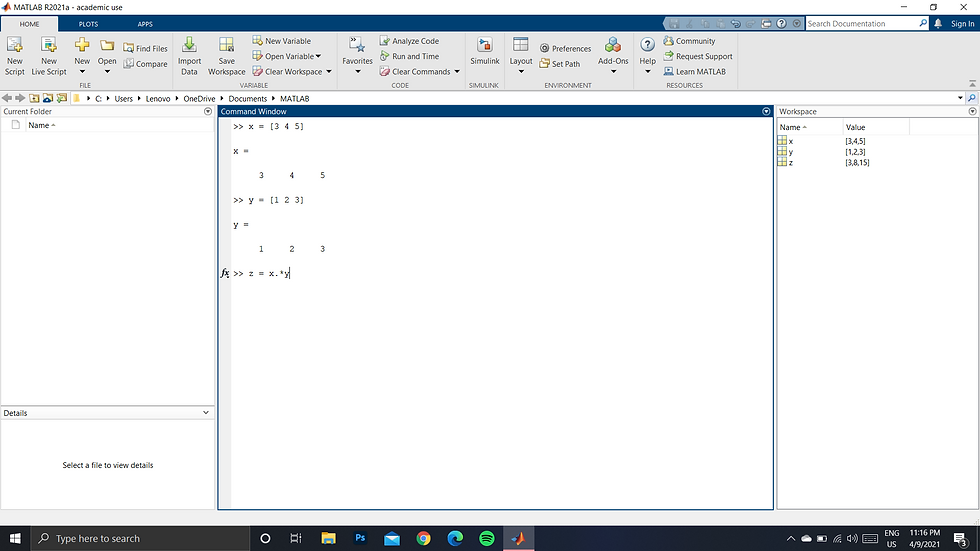top of page# Multiply matrices corresponding to its elements in MATLAB

1. Open your command window in MATLAB2. Enter the first matrix, say, x = [3 4 5 ]3. Enter another matrix, say, y = [1 2 3] corresponding to which you want to multiply.4. Then type a new variable, say, z = x.*y, using a "dot" will make sure that corresponding element multiplication is done rather than the conventional method.5. Hit enter